# Introduction to tests for data scientists

#### Sarah Diot-Girard

• Data scientist at• Interested in devops and ethics
•@SdgJlbl

## Prologue

### Why test?

Testing by users is no longer a thing

### Why test?

ML algorithms fail silently

### Why test?

Bugs in data + bugs in code = a lot of bugs

### What is a test?

A piece of software
that can either pass or fail
and whose outcome is correlated
with functional correctness

### What is a good test?

• Thorough
• Specific• Repeatable (stable)
• Isolated
• Fast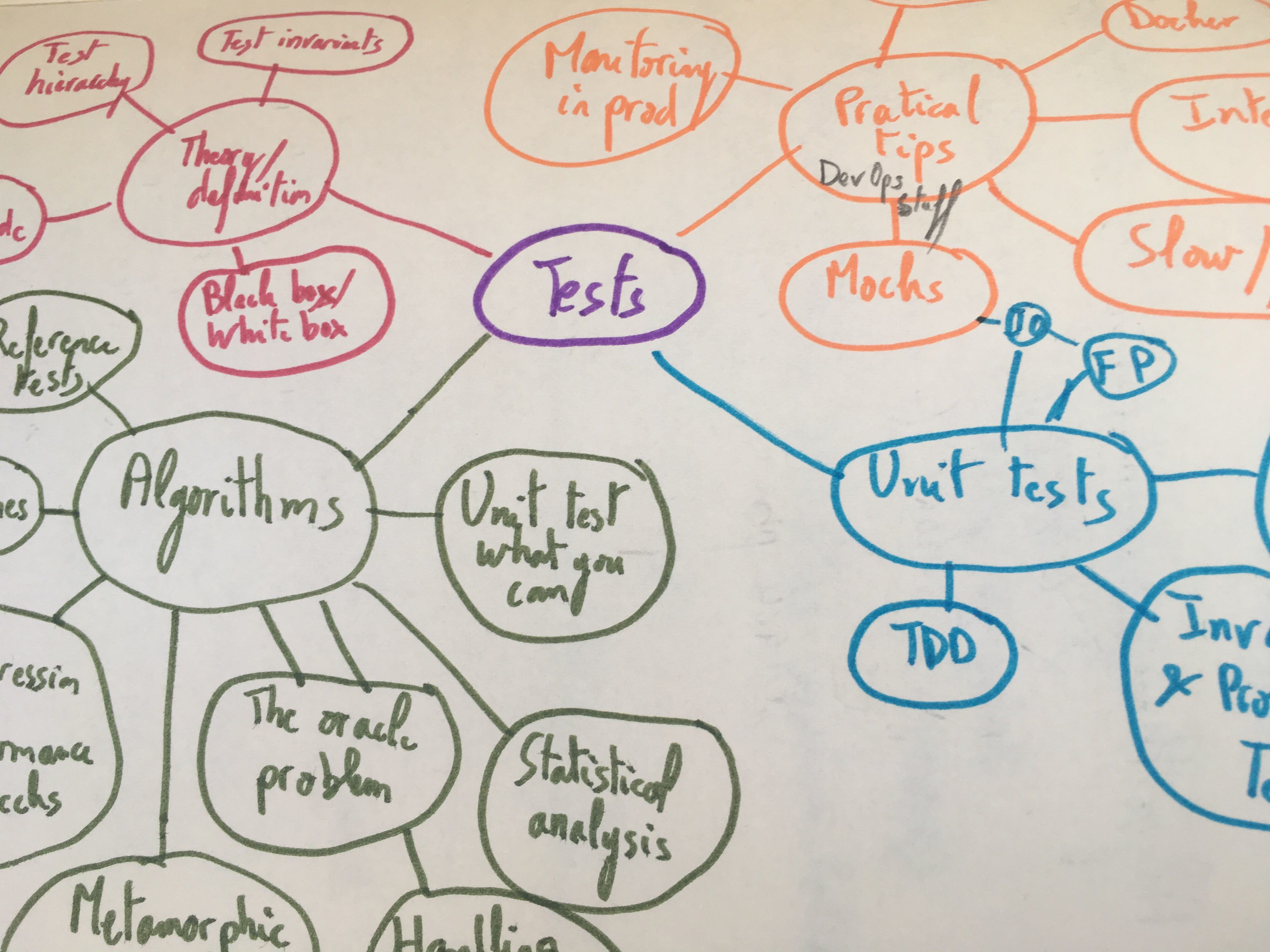### Topics we won't cover

• How to set up a CI
• Data monitoring
• Test-driven development
• Coverage and mutation testing

### Topics we will cover

Testing a ML pipeline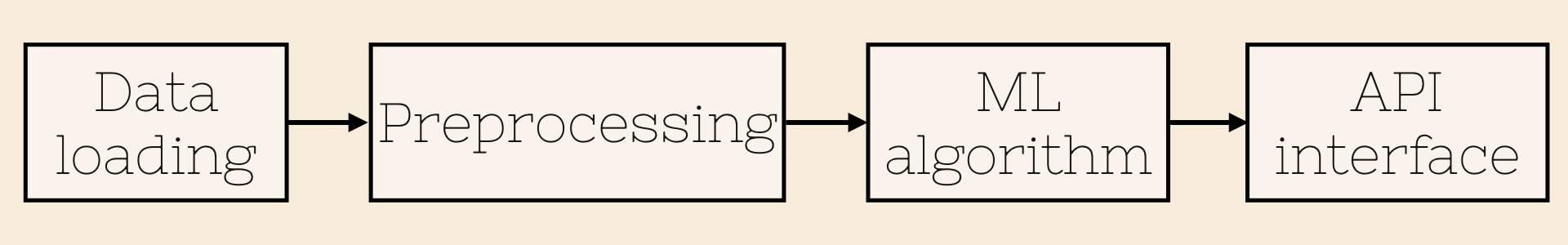## Chapter 1

### Unit tests for preprocessing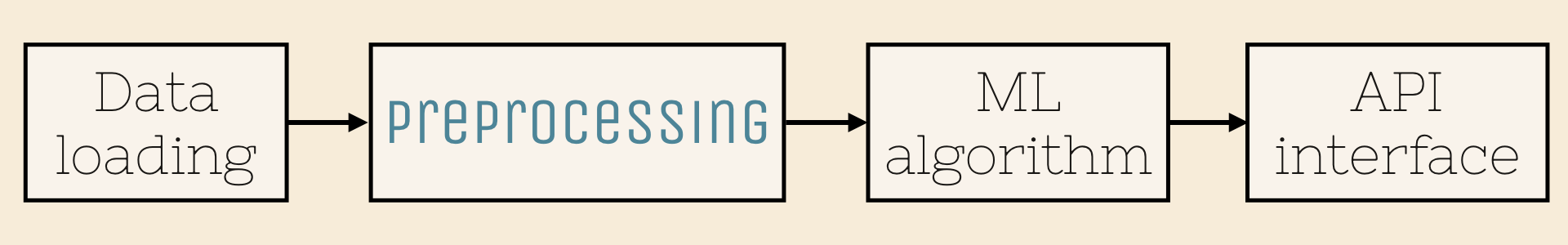### Preprocessing

• Handling missing values
• Encoding labels
• Normalizing values
• ...

### Preprocessing

All these operations can be written as functions.


df_filled = fill_missing_values(df_raw, how='average')

df_cleaned = remove_outliers(df_filled)


### Functional programming

y = f(x)

-> no side effects


df = fill_missing_values(df_raw, how='average') ✔

fill_missing_values(df_raw, how='average') ✗

df = fill_missing_values('raw_df.csv', how='average') ✗

df_out, label_mapping = encode_labels(df_in)  ✔


Output(s) depend only on input(s)

### Unit tests

... test units of source code

### Unit tests

... work great with functional programming

### Unit tests

Property-based testing -> hypothesis

import numpy as np
from hypothesis import given
from hypothesis.extra.numpy import arrays

def normalize(arr):
return np.zeros(arr.shape)

@given(arrays(dtype=float, shape=(3,4)))
def test_normalize_is_between_bounds(input):
output = normalize(input)
assert np.all(output >= 0)
assert np.all(output <= 1)

@given(arrays(dtype=float, shape=(3,4)))
def test_normalize_is_idempotent(a):
normalized_input = normalize(input)
output = normalize(normalized_input)
assert np.all(output == normalized_input)

Add a few trivial tests with simple examples

## Chapter 2

### ML algorithms and their plight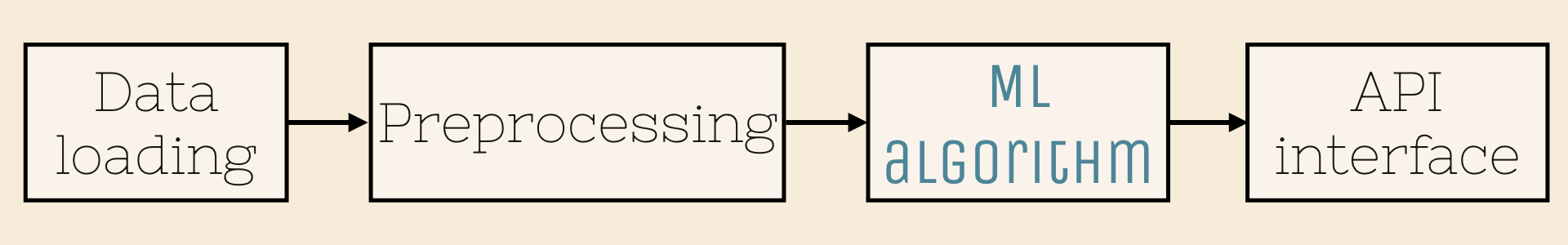### Algorithms

are hard to unit test

### Algorithms

• ... are iterative processes
• ... are stochastic
• ... suffer from the oracle problem
• ... are slow

### Algorithms are iterative

Unit-test what you can

### Component tests

... test whole chunks of your code

### Algorithms are stochastic

Fix random seed

Average multiple runs

### Algorithms don't have a test oracle

Differential testing

Metamorphic testing

Reference tests

### Differential testing

Check multiple implementations
against one another

### Differential testing

Check for non-regression between

Check against a random or uniform baseline

### Metamorphic testing

Run the same implementation multiple times with modified input

$\Phi (f(x)) = f( \phi (x))$

### Metamorphic testing


def test_compute_variance():

x = np.random.rand(25)

assert compute_variance(x * -1) == compute_variance(x)

assert compute_variance(2 * x) == 4 * compute_variance(x)


### Reference tests

Store input and reference output

Check that computed output matches reference output

### The necessary evil of reference tests

• Low coverage
• Hard to maintain

### Reference tests

... can be kept up-to-date with tdda

### Algorithms are slow

And many mitigation strategies make them slower

Have two test suites:

• a fast one that runs at each modification
• a slow one running every night

## Chapter 3

### I/O and end-to-end tests### How to test I/O?

Functional programming won't work
because of side-effect

Mocking can help

### Mocks

... mimic the behaviour of an object in a controlled way


def preprocessing(df: pd.DataFrame) -> pd.DataFrame:
...

def model_training(arr: np.ndarray):
...


End-to-end tests

### Test hierarchy### Test hierarchy### Test hierarchy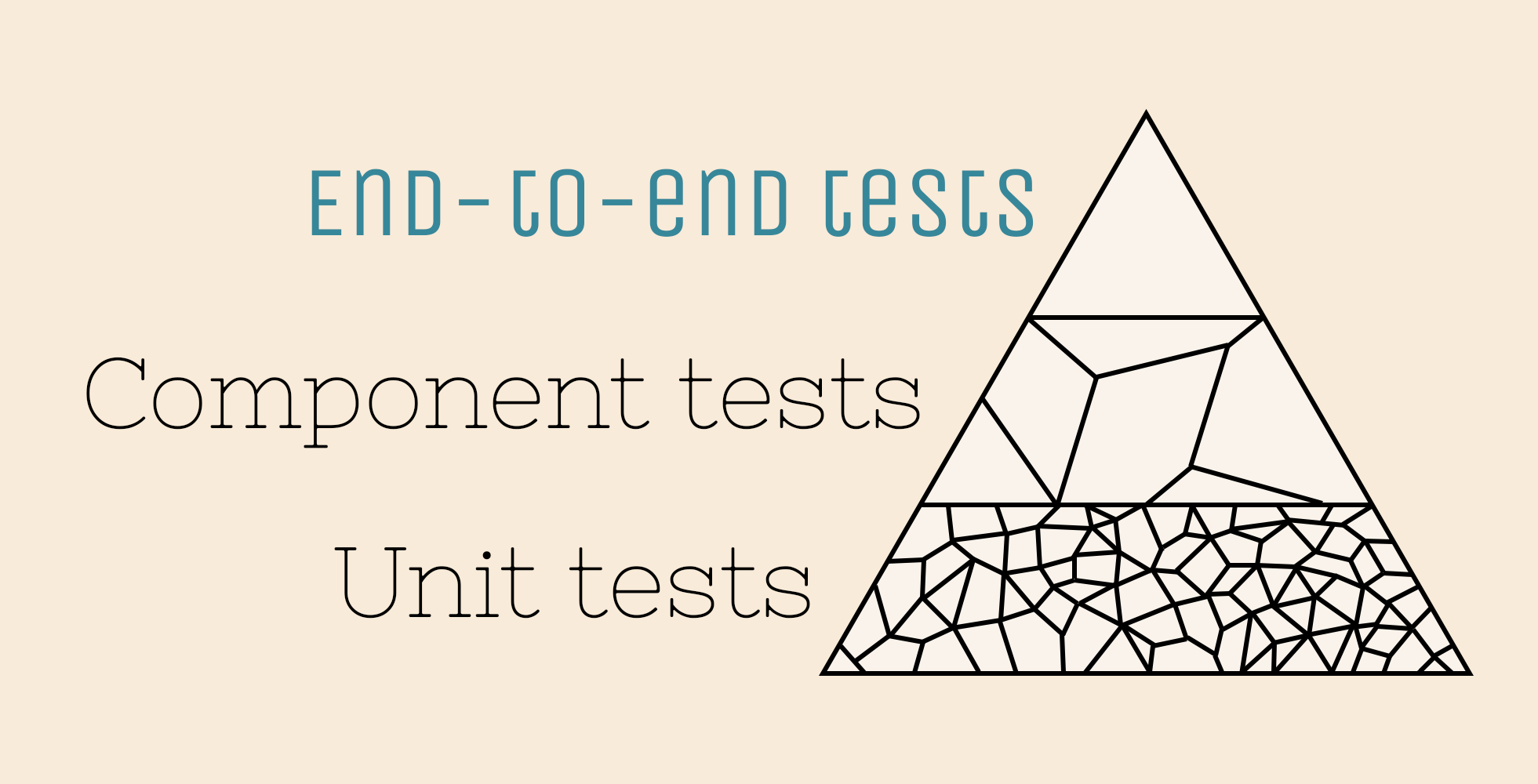### Test hierarchy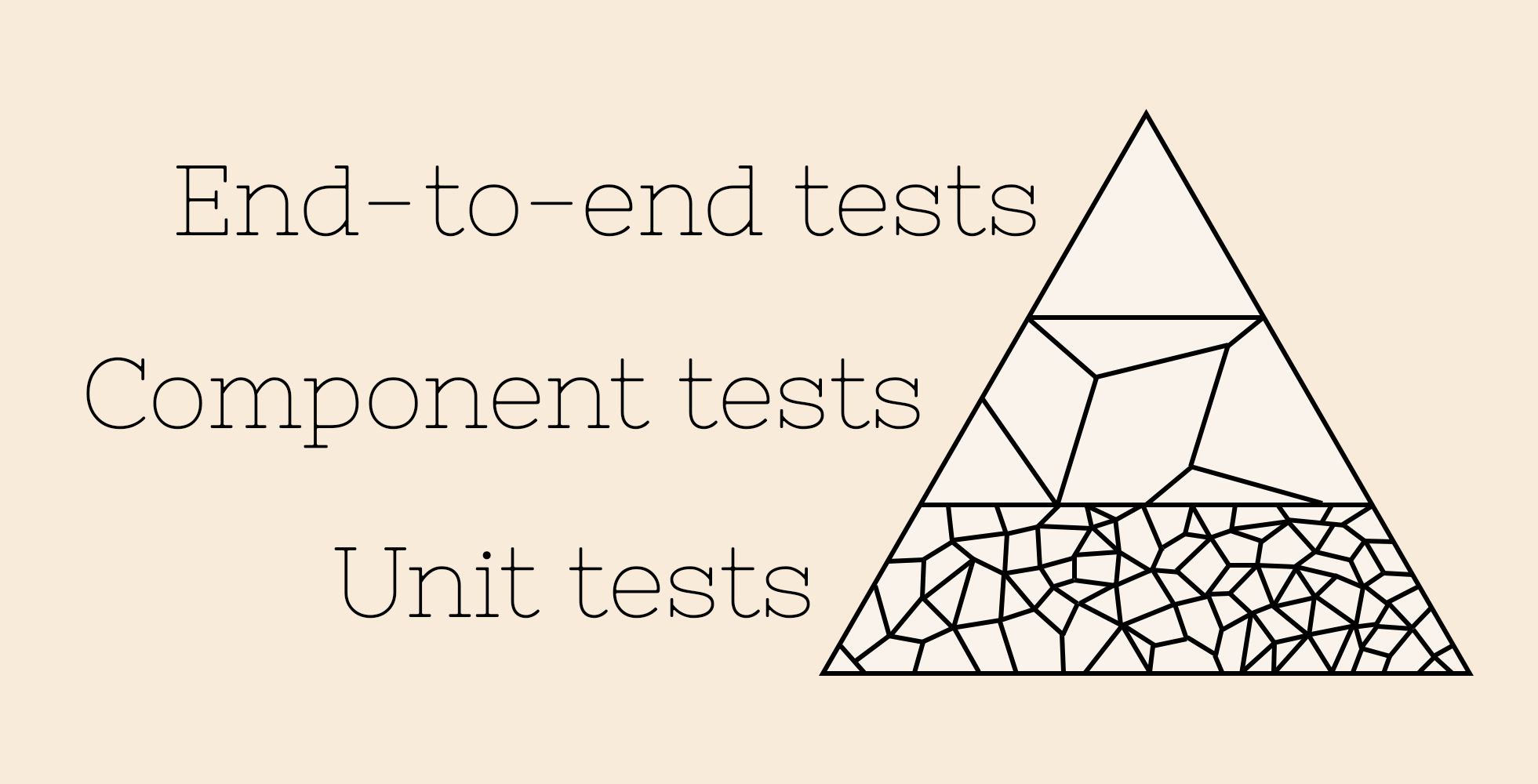### Setting up a CI

Automated tests are really automatic when they are automatically run

## Epilogue

### Tips and tricks for better testing of ML pipelines

#### Have some automated tests

Anything is better than nothing

#### Have a CI

Else your tests won't run as often
as they should

But not hopeless

#### Befriend software engineers

They are happy to share their knowledge

### That's all for me@SdgJlbl### To go further

Testing and validating machine learning classifiers by metamorphic testing, X. Xie et al

On testing machine learning programs, H. Ben Braieka and F. Khomh

Documentation of hypothesis

Documentation of tdda